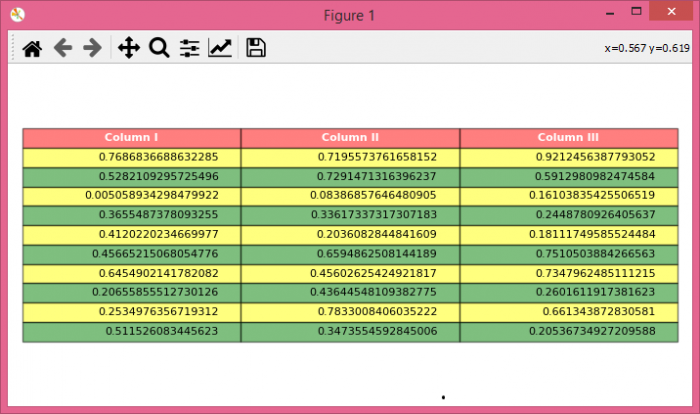# How to change the transparency/opaqueness of a Matplotlib Table?

To change the transparency/opaqueness of a matplotlib table, we can atke following steps

## Steps

• Set the figure size and adjust the padding between and around the subplots.

• Create a figure and a set of subplots.

• Create a random dataset with 10×3 dimension.

• Create a tuple of columns.

• Get rid of the axis markers using axis('off').

• Create a table with data and columns.

• Iterate each cell of the table and change its transparency/opaqueness using set_alpha() method.

• To display the figure, use Show() method.

## Example

import numpy as np
from matplotlib import pyplot as plt

plt.rcParams["figure.figsize"] = [7.00, 3.50]
plt.rcParams["figure.autolayout"] = True

fig, axs = plt.subplots(1, 1)
data = np.random.random((10, 3))
columns = ("Column I", "Column II", "Column III")

axs.axis('off')

the_table = axs.table(cellText=data, colLabels=columns, loc='center')

for k, cell in the_table._cells.items():
cell.set_edgecolor('black')
if k == 0 or k < 0:
cell.set_text_props(weight='bold', color='w')
cell.set_facecolor('red')
cell.set_alpha(0.5)
else:
cell.set_facecolor(['green', 'yellow'][k % 2])
cell.set_alpha(0.5)

plt.show()


## Output

It will produce the following output −